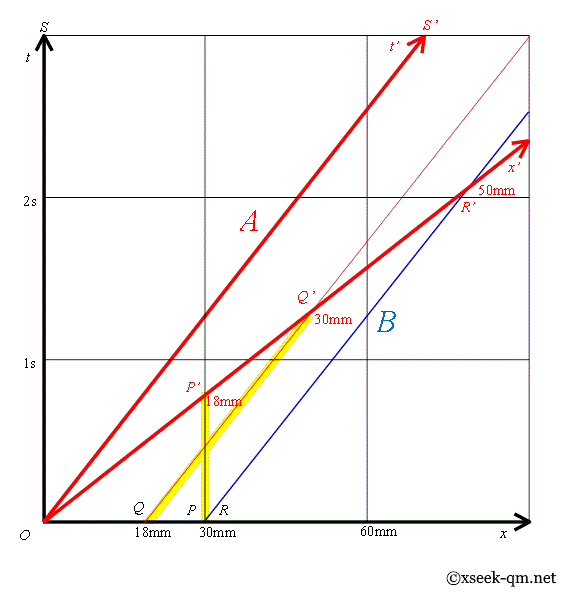2015/01/12
Published 2002/3/8

I will introduce a Bell's spaceship paradox.

Physicist E. Dewan and M. Beran published "two spaceships paradox" in 1959. It is called "Bell's spaceship paradox" because Physicist J. S. Bell introduced it in 1987.

Generally, we explain the thought experiment by two spaceships. However, it is difficult to imagine the thought experiment because the speed of light is too fast. Then, we suppose that the speed of light is 30 millimeters per second.

Now, there is a caterpillar of a butterfly on a desk. The length of the body is 30 millimeters.The caterpillar accelerated for 10 seconds. The velocity became 24 millimeters per second. How long does the length of the body of the caterpillar become?

The formula of the Lorentz contraction is shown below.The variables are L0 = 30mm, v = 24mm/s, and c = 30mm/s. We calculate the Lorentz contraction as follows.Therefore, we have L = 18mm. The length of the body of the caterpillar contracts by the Lorentz contraction.

However, the length of the body of the caterpillar is 30 millimeters in the kinetic system S ' of the caterpillar.

Then we put two ants on the desk. The length between two ants is 30 millimeters.The two ants accelerated for 10 seconds. The two ants started accelerating at the same time in the rest system S. Then, the two ants stopped accelerating at the same time in the rest system S. The velocity of the two ants became 24 millimeters per second. How long is the length between two ants in the rest system S?

Some person might think that the length between two ants contracts by the Lorentz contraction. However, the length between two ants does not contract in the rest system S because the stopping time of the acceleration of the two ants is same in the rest system S.

If we moderately adjust the acceleration of ants, the ants can accelerate by the acceleration a=2.4mm/s2 for 10 seconds in the stationary system S. We suppose that the position of the ant A at the time 0 second is 0 millimeters. Then, we are able to express the position x(t) of the ant A at the time t seconds in the following formula.We obtain the position the ant A after 10 seconds by calculating the above formula. It is 120 millimeters. On the other hand, if we suppose that the position of the ant B at the time 0 second is 30 millimeters. we are able to express the position y(t) of the ant B at the time t seconds in the following formula.We obtain the position the ant B after 10 seconds by calculating the above formula. It is 150 millimeters. Therefore, the interval between two ants is 30 millimeters after 10 seconds. The velocities of two ants are 24mm/s. Two ants keep the velocity after that. Therefore, the interval of the two ants is always 30 millimeters in the stationary system S.

Then, a thread hangs between two ants. What happens when the two ants started accelerating?

The thread breaks when the two ants started accelerating. Some person might not believe in the result because the length between two ants does not contract in the rest system S. Why does the thread break?We consider the kinetic system S ' of the ant A. The simultaneity of the ant A changes when the ant A started accelerating. Therefore, the ant B finishes the acceleration earlier than the ant A. Then, the velocity of the ant B is faster than the velocity of the ant A. Therefore, the length between two ants gets longer when the ant A finishes the acceleration. The length becomes 50 millimeters in the kinetic system S ' of the ant A. Therefore, the thread breaks.

It is mysterious phenomena in the rest system S because the length of the thread does not change in the rest system S. Why does the thread break? Actually, the thread breaks because the distance of the intermolecular force gets shorter in the rest system S.

## Minkowski space

I would like to explain the Lorentz contraction by the Minkowski space.The Lorentz transformation is shown below.Here, we defined the variable γ and β as follows.The variables are v = 24mm/s, and c = 30mm/s. We calculate the Lorentz transformation as follows.The world line of the ant A is line A. The world line of the ant B is line B. The length between two ants is the segment OR of the line in the rest system S. The length between two ants is the segment OR ' of the line in the kinetic system S '.

The length of the body of the caterpillar is the segment OQ of the line in the rest system S. The length of the body of the caterpillar is the segment OQ ' of the line in the kinetic system S '.

I would like to focus on the cross structure of the segment PP ' of the line and the segment QQ ' of the line. I guess the cross structure is the essential structure of the Lorentz transformation.

Related Articles: